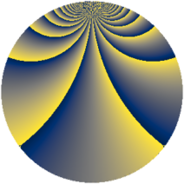# Properties

 Label 1110.2.bqLevel $1110$ Weight $2$ Character orbit 1110.bq Rep. character $\chi_{1110}(49,\cdot)$ Character field $\Q(\zeta_{18})$ Dimension $240$ Sturm bound $456$

# Related objects

## Defining parameters

 Level: $$N$$ $$=$$ $$1110 = 2 \cdot 3 \cdot 5 \cdot 37$$ Weight: $$k$$ $$=$$ $$2$$ Character orbit: $$[\chi]$$ $$=$$ 1110.bq (of order $$18$$ and degree $$6$$) Character conductor: $$\operatorname{cond}(\chi)$$ $$=$$ $$185$$ Character field: $$\Q(\zeta_{18})$$ Sturm bound: $$456$$

## Dimensions

The following table gives the dimensions of various subspaces of $$M_{2}(1110, [\chi])$$.

Total New Old
Modular forms 1416 240 1176
Cusp forms 1320 240 1080
Eisenstein series 96 0 96

## Trace form

 $$240q + O(q^{10})$$ $$240q + 12q^{14} - 12q^{19} + 24q^{21} - 24q^{25} - 24q^{26} - 24q^{30} + 72q^{31} - 36q^{34} - 96q^{35} + 240q^{36} + 168q^{41} - 12q^{44} + 60q^{46} - 72q^{49} + 24q^{50} - 84q^{55} + 144q^{59} - 72q^{61} + 120q^{64} + 12q^{65} - 24q^{69} + 12q^{70} - 144q^{71} + 24q^{74} + 12q^{76} + 24q^{79} + 24q^{84} + 72q^{85} - 24q^{86} + 12q^{89} + 180q^{91} + 12q^{94} + 60q^{95} - 12q^{99} + O(q^{100})$$

## Decomposition of $$S_{2}^{\mathrm{new}}(1110, [\chi])$$ into newform subspaces

The newforms in this space have not yet been added to the LMFDB.

## Decomposition of $$S_{2}^{\mathrm{old}}(1110, [\chi])$$ into lower level spaces

$$S_{2}^{\mathrm{old}}(1110, [\chi]) \cong$$ $$S_{2}^{\mathrm{new}}(185, [\chi])$$$$^{\oplus 4}$$$$\oplus$$$$S_{2}^{\mathrm{new}}(370, [\chi])$$$$^{\oplus 2}$$$$\oplus$$$$S_{2}^{\mathrm{new}}(555, [\chi])$$$$^{\oplus 2}$$